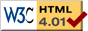Nav: [home] > [ani] > [utils] > [genpoly]

## GenPoly -- Generating Polynomials

### Description

GenPoly is a simple program to generate polynomials with certain properties.
Note: I am talking about polynomials and not splines. GenPoly can create polynomials of any order (dependent on the number of points you specify). However, polynomials are not as easy to handle as splines. If you ever tried to create a nice polynomial with certain properties you know what I mean: The spline joins some points in a natural and smooth way; the polynomial goes through the points, too, but it may need to have large extrema in between them.
Sedgewick made some dark allusions about that but I did not believe it before actually seeing it myself. So, try out but don't be surprised by strange results.

Some maths:
Weierstrass (1815--1897) proved that it is possible to approximate any steady function (in a compact interval) using polynomials. This means, he proved that for a given tolerance, a polynomial exists which does not vary from the original function by an amount larger than the tolerance.

Finding such a polynomial is not trivial however. You can take samples from the original function and make the polynomial go through these points but the result will look completely different than the original function. (This is what I meant above.)
That is why people are using splines and why polynomials are of limited use.
Note, however, that you can get a fairly well polynomial approximation of a given function using Tschebyschoff interpolation. (That method chooses itself where to take samples for the interpolation.)

I provide only the source code but compiling it should be trivial.

 Source: genpoly.cpp   [10kb C++ source] Version: 0.2   (2004-03-25) Author: Wolfgang Wieser   (report bugs here) License: GNU GPL (Version 2)

Requirements:
Just a C++ compiler (GCC recommended).

Platform:
GenPoly works fine on my Linux-ix86 box but it should be fairly platform-independent. It may even compile on Window\$ without tweaking.

Compiling:
Just call gcc for the one source file:
gcc genpoly.cpp -o genpoly -lm -fno-rtti -fno-exceptions -O2

Install:
Simply copy the genpoly to the location you desire.

Bugs:
The matrix inversion is probably numerically instable. I have the impression that it worked fine, though.
Other bugs may be reported to me (email on bottom of this page and in source file).

### GenPoly Manual

GenPoly is a command line utility. You specify some properties of the polynomial on the command line and hit enter to have the polynomial calculated and dumped to the terminal.
GenPoly understands the following command line options:

--help
 Print some usage information.

-c
 Print polynomial coefficients only.

-p
 Use pow(x,y) in output instead of x^y. Useful if you want to insert the polynomial into C/C++ code or POVRay scenes.

-a
 Use x**y instead of x^y. Useful when you want to plot the resulting polynomial using GnuPlot.

-q
 Quiet operation.

The following command line options (without leading dash) can be used to specify the polynomial properties:
Remember to use quotes to prevent the shell from interpreting the brackets.

p(x,y)
 Make the polynimial go through the point at position (x,y).

a(x,m)
 Make the polynimial have ascent m at X-position x.

e(x)
 Make the polynimial have a first-order extremum at X-position x.

e(x,y)
 Make the polynimial have a first-order extremum at position (x,y).

w(x)
 Make the polynimial have a first-order wending point at X-position x.

w(x,y)
 Make the polynimial have a first-order wending point at position (x,y).

i(x,y,a)
 This is the general property specification, all those above are just special shorthands of this one: Specify that the polynomial's a-th derivation at X-position x shall have the value y.